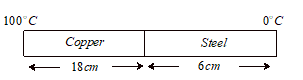Conduction
Question

# The coefficient of thermal conductivity of copper is 9 times that of steel. In the composite cylindrical bar shown in the figure. What will be the temperature at the junction of the copper and steel.Easy
Solution

## Rate of heat flow far both the rods are same.$\frac{\mathrm{Q}}{\mathrm{t}}=\mathrm{KA}\left(\frac{{\mathrm{\theta }}_{1}-{\mathrm{\theta }}_{2}}{\mathrm{l}}\right)=\text{constant}$$\frac{9\mathrm{K}\left(100-\mathrm{\theta }\right)}{18}=\frac{\mathrm{K}\left(\mathrm{\theta }-0\right)}{6}$$300-3\mathrm{\theta }=\mathrm{\theta }$$\mathrm{\theta }={75}^{\mathrm{o}}\mathrm{C}$

Get Instant Solutions